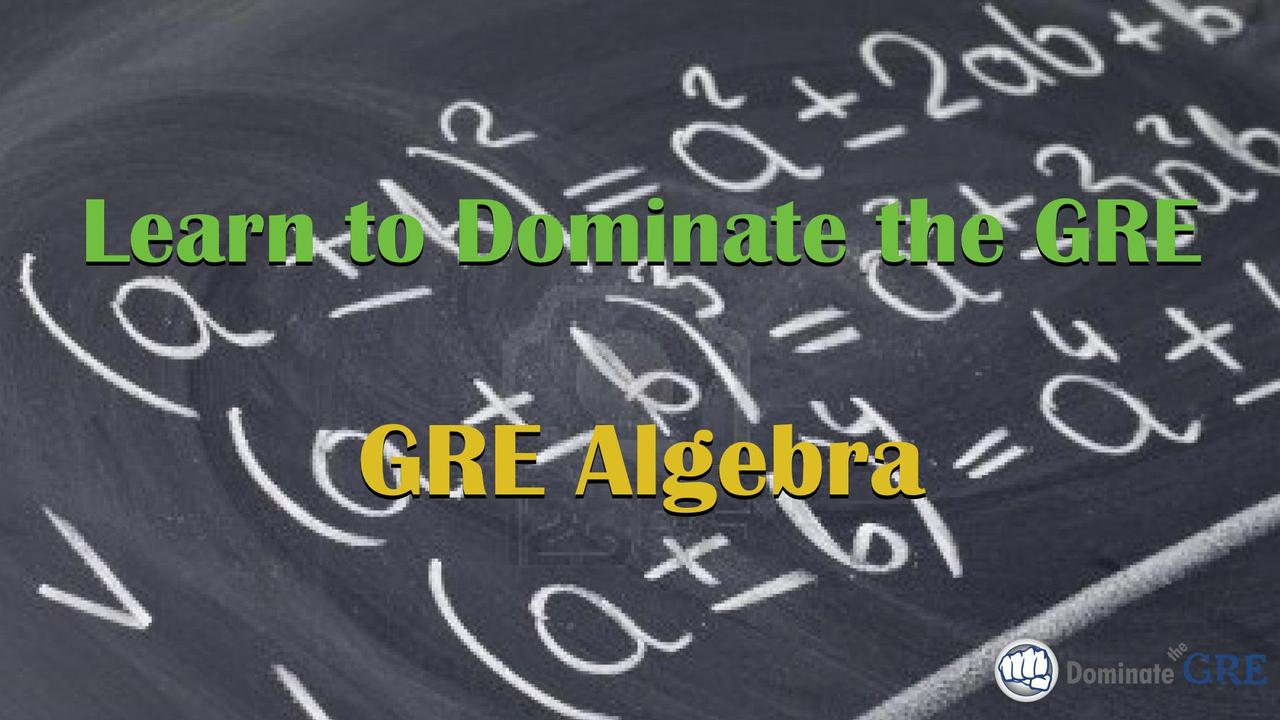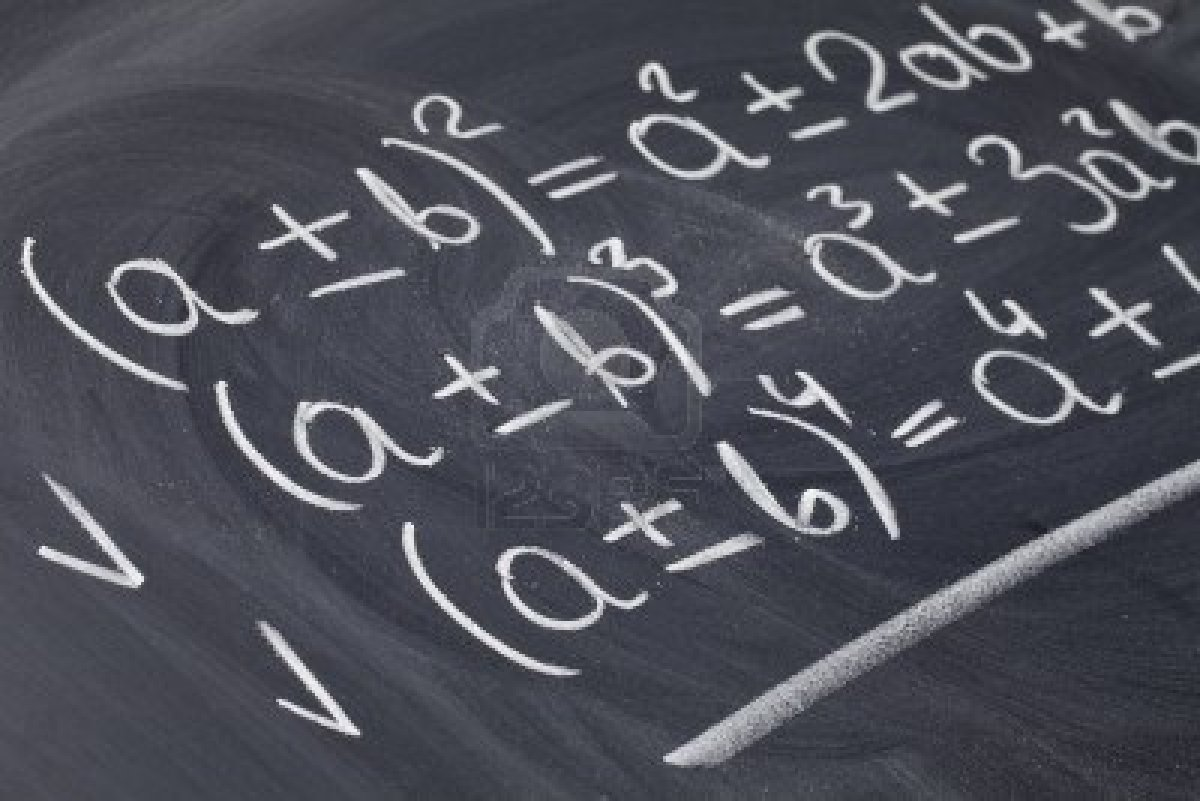# GRE Algebra - Video CourseWe go to great lengths at Dominate the GRE to help you avoid doing difficult algebra on the GRE. There are useful non-standard math techniques, for example, that can help you make variables your friend and turn otherwise problematic word problems into run-of-the-mill math problems.

Sometimes, however, solving algebra problems on the GRE is simply unavoidable.

In this course you will learn how to tackle several of the most commonly-tested “difficult” algebra concepts tested on the GRE including quadratic equations, simultaneous equations, and inequalities. We'll also cover GRE algebra questions that involve powers (exponents) and roots (radicals) as well as algebraic expressions that you may need to either simplify or manipulate in some way. But don’t worry — there won’t be a lot of long formulas to memorize (we still remember a song our 7th grade algebra teacher taught us to help us remember the Quadratic Formula, and don’t worry, we won’t be signing it to you here!); rather, we’ll focus on teaching you how the GRE tests each of these topics and equip you with plenty of examples to illustrate everything you’ll need to master these GRE algebra problem types and get more right answers on test day.

### Specifically, the GRE video tutorials in this module cover:

• Simultaneous equations, with multiple approaches and strategies so that you can choose the method that works best for you;
• GRE quadratic equations, with an emphasis on the three most commonly-tested quadratic equations on the GRE;
• The FOIL Method, including how to factor quadratic equations using the “reverse FOIL” method;
• Figuring out how many roots a quadratic equation has and comparing those roots for determining relative size on GRE Quantitative Comparison questions;
• The in's and out's of inequalities;
• GRE exponents, including combining exponents by addition/subtraction and multiplication/division as well as a fool-proof strategy for avoiding the most common exponent traps found on the GRE;
• Detailed review of roots and radicals;
• The most commonly-tested exponents and square roots on the GRE;
• Simplifying algebraic expressions;
• Algebraic functions;
• Numerous application examples to fully illustrate all of the concepts taught in this course;
• And more!

Oh, and did we mention that this course includes a practice worksheet that will give you the opportunity to apply what you learn to real sample GRE algebra questions? Each question of the worksheet comes with a detailed video answer explanation showing you the most efficient way to solve it.

So what are you waiting for? Take your GRE preparation to the next level. Add this course to your library now and prepare to dominate the GRE!